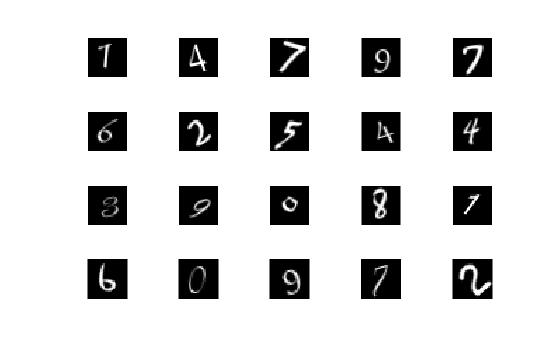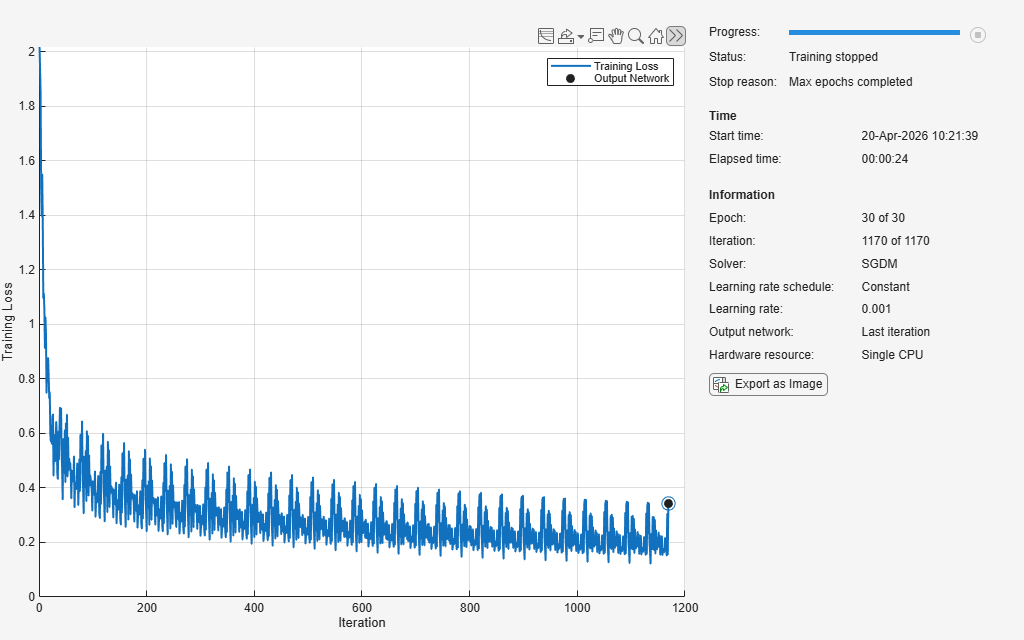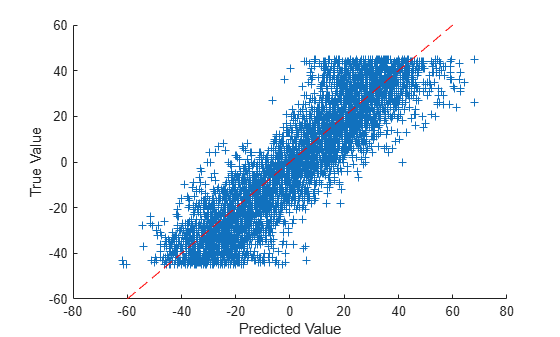# Convert Classification Network into Regression Network

This example shows how to convert a trained classification network into a regression network.

Pretrained image classification networks have been trained on over a million images and can classify images into 1000 object categories, such as keyboard, coffee mug, pencil, and many animals. The networks have learned rich feature representations for a wide range of images. The network takes an image as input, and then outputs a label for the object in the image together with the probabilities for each of the object categories.

Transfer learning is commonly used in deep learning applications. You can take a pretrained network and use it as a starting point to learn a new task. This example shows how to take a pretrained classification network and retrain it for regression tasks.

The example loads a pretrained convolutional neural network architecture for classification, replaces the layers for classification and retrains the network to predict angles of rotated handwritten digits. Optionally, you can use `imrotate` (Image Processing Toolbox™) to correct the image rotations using the predicted values.

Load the pretrained network from the supporting file `digitsNet.mat`. This file contains a classification network that classifies handwritten digits.

```load digitsNet layers = net.Layers```
```layers = 15x1 Layer array with layers: 1 'imageinput' Image Input 28x28x1 images with 'zerocenter' normalization 2 'conv_1' Convolution 8 3x3x1 convolutions with stride [1 1] and padding 'same' 3 'batchnorm_1' Batch Normalization Batch normalization with 8 channels 4 'relu_1' ReLU ReLU 5 'maxpool_1' Max Pooling 2x2 max pooling with stride [2 2] and padding [0 0 0 0] 6 'conv_2' Convolution 16 3x3x8 convolutions with stride [1 1] and padding 'same' 7 'batchnorm_2' Batch Normalization Batch normalization with 16 channels 8 'relu_2' ReLU ReLU 9 'maxpool_2' Max Pooling 2x2 max pooling with stride [2 2] and padding [0 0 0 0] 10 'conv_3' Convolution 32 3x3x16 convolutions with stride [1 1] and padding 'same' 11 'batchnorm_3' Batch Normalization Batch normalization with 32 channels 12 'relu_3' ReLU ReLU 13 'fc' Fully Connected 10 fully connected layer 14 'softmax' Softmax softmax 15 'classoutput' Classification Output crossentropyex with '0' and 9 other classes ```

The data set contains synthetic images of handwritten digits together with the corresponding angles (in degrees) by which each image is rotated.

Load the training and validation images as 4-D arrays using `digitTrain4DArrayData` and `digitTest4DArrayData`. The outputs `YTrain`` `and `YValidation` are the rotation angles in degrees. The training and validation data sets each contain 5000 images.

```[XTrain,~,YTrain] = digitTrain4DArrayData; [XValidation,~,YValidation] = digitTest4DArrayData;```

Display 20 random training images using `imshow`.

```numTrainImages = numel(YTrain); figure idx = randperm(numTrainImages,20); for i = 1:numel(idx) subplot(4,5,i) imshow(XTrain(:,:,:,idx(i))) drawnow end```### Replace Final Layers

The convolutional layers of the network extract image features that the last learnable layer and the final classification layer use to classify the input image. These two layers, `'fc'` and `'classoutput'` in `digitsNet`, contain information on how to combine the features that the network extracts into class probabilities, a loss value, and predicted labels. To retrain a pretrained network for regression, replace these two layers with new layers adapted to the task.

Replace the final fully connected layer, the softmax layer, and the classification output layer with a fully connected layer of size 1 (the number of responses) and a regression layer.

```numResponses = 1; layers = [ layers(1:12) fullyConnectedLayer(numResponses) regressionLayer];```

### Freeze Initial Layers

The network is now ready to be retrained on the new data. Optionally, you can "freeze" the weights of earlier layers in the network by setting the learning rates in those layers to zero. During training, `trainNetwork` does not update the parameters of the frozen layers. Because the gradients of the frozen layers do not need to be computed, freezing the weights of many initial layers can significantly speed up network training. If the new data set is small, then freezing earlier network layers can also prevent those layers from overfitting to the new data set.

Use the supporting function `freezeWeights` to set the learning rates to zero in the first 12 layers.

`layers(1:12) = freezeWeights(layers(1:12));`

### Train Network

Create the network training options. Set the initial learn rate to 0.001. Monitor the network accuracy during training by specifying validation data. Turn on the training progress plot, and turn off the command window output.

```options = trainingOptions('sgdm',... 'InitialLearnRate',0.001, ... 'ValidationData',{XValidation,YValidation},... 'Plots','training-progress',... 'Verbose',false);```

Create the network using `trainNetwork`. This command uses a compatible GPU if available. Otherwise, `trainNetwork` uses the CPU. A CUDA®-enabled NVIDIA® GPU with compute capability 3.0 or higher is required for training on a GPU.

`net = trainNetwork(XTrain,YTrain,layers,options);`### Test Network

Test the performance of the network by evaluating the accuracy on the validation data.

Use `predict` to predict the angles of rotation of the validation images.

`YPred = predict(net,XValidation);`

Evaluate the performance of the model by calculating:

1. The percentage of predictions within an acceptable error margin

2. The root-mean-square error (RMSE) of the predicted and actual angles of rotation

Calculate the prediction error between the predicted and actual angles of rotation.

`predictionError = YValidation - YPred;`

Calculate the number of predictions within an acceptable error margin from the true angles. Set the threshold to be 10 degrees. Calculate the percentage of predictions within this threshold.

```thr = 10; numCorrect = sum(abs(predictionError) < thr); numImagesValidation = numel(YValidation); accuracy = numCorrect/numImagesValidation```
```accuracy = 0.7532 ```

Use the root-mean-square error (RMSE) to measure the differences between the predicted and actual angles of rotation.

` rmse = sqrt(mean(predictionError.^2))`
```rmse = single 9.0270 ```

### Correct Digit Rotations

You can use functions from Image Processing Toolbox to straighten the digits and display them together. Rotate 49 sample digits according to their predicted angles of rotation using `imrotate` (Image Processing Toolbox).

```idx = randperm(numImagesValidation,49); for i = 1:numel(idx) I = XValidation(:,:,:,idx(i)); Y = YPred(idx(i)); XValidationCorrected(:,:,:,i) = imrotate(I,Y,'bicubic','crop'); end```

Display the original digits with their corrected rotations. Use `montage` (Image Processing Toolbox) to display the digits together in a single image.

```figure subplot(1,2,1) montage(XValidation(:,:,:,idx)) title('Original') subplot(1,2,2) montage(XValidationCorrected) title('Corrected')```